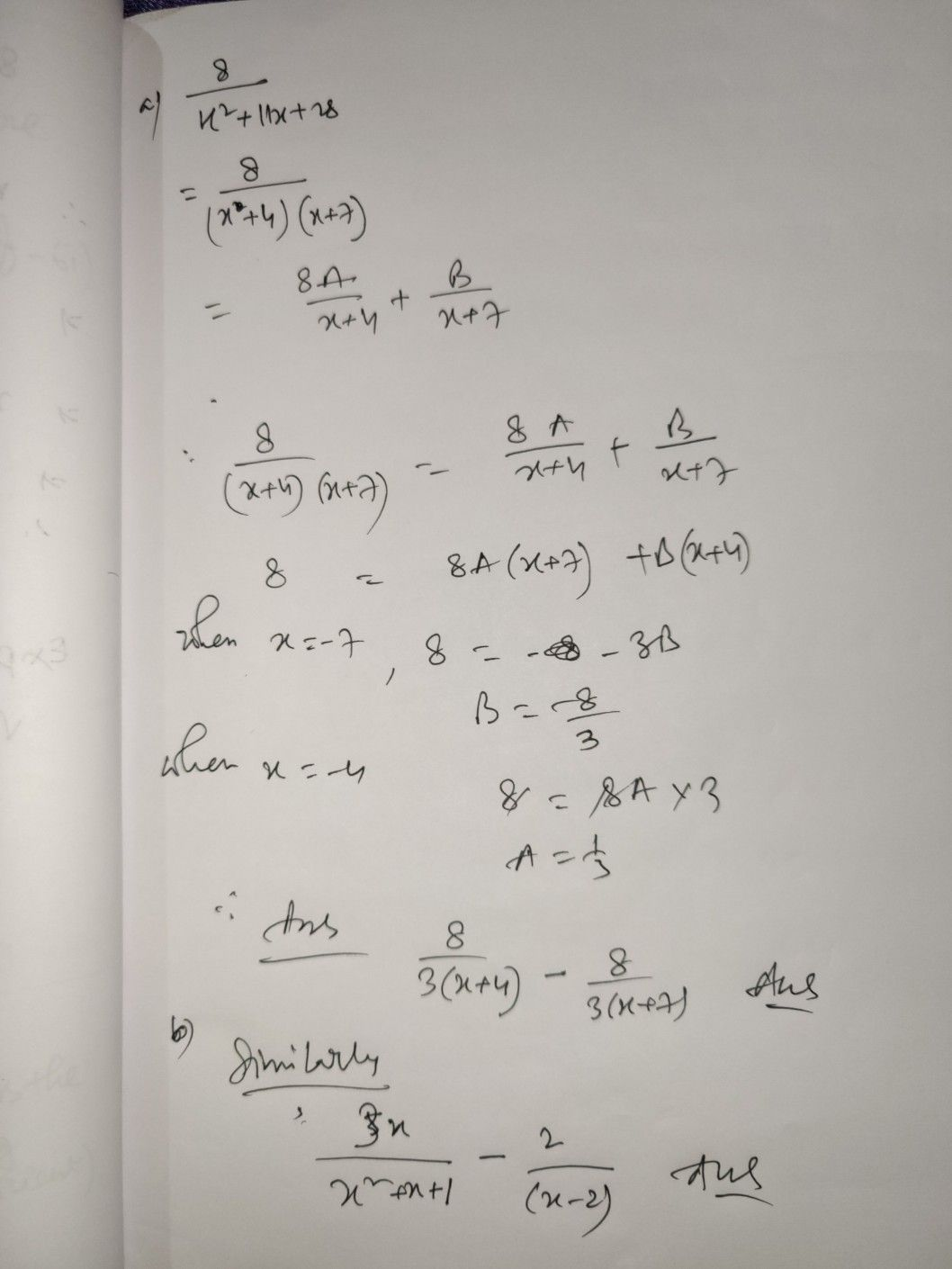Symbol
Problem$3$ Express the following as a sum of partial fractions. $a\right)$ $\dfrac {8} {x^{2}+11x+28}$ $5x$ $b\right)$ $\bar{\left(x^{2}+x+1\left(x-2\right)}$